# Venn digram | Operation on sets

## Universal Set

A Universal is the set of all elements under consideration, denoted by capital U.

## Venn diagram

• Venn diagrams were introduced in 1880 by John Venn (1834-1923).
• These diagrams consist of rectangles and closed curves usually circles.
• The universal set is represented usually by a rectangle and its subsets by circles.
• Venn diagrams normally comprise overlapping circles. The interior of the circle symbolically represents the elements of the set, while the exterior represents elements that are not members of the set. For instance, in a two-set Venn diagram, one circle may represent the group of all wooden objects, while another circle may represent the set of all tables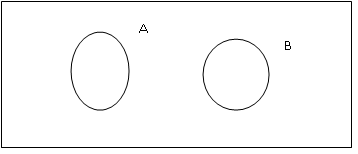## Operation on Sets

### Union of Sets

The union of two sets $A$ and $B$ is the set $C$ which consists of all those elements which are either in $A$ or in $B$ (including those which are in both). In symbols, we write.
$A \cup B = \left\{ {x:x \in A{\rm{\; or \;}}x \in B} \right\}$

#### Venn Digram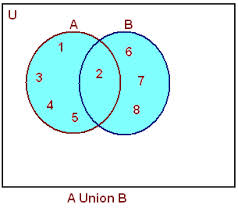#### Some Properties of the Operation of Union

• Commutative law : $X \cup Y = Y \cup X$
• Associative law : $\left( {X \cup Y} \right) \cup Z = X \cup \left( {Y \cup Z} \right)$
• Law of identity element, $\phi$ is the identity of $\cup$ : $X \cup \phi = X$
• Idempotent law : $X \cup X = X$
• Law of U : $U \cup X = U$

### Intersection of Sets

The Intersection of two sets $A$ and $B$ is the set $C$ which consists of all those elements which are present in both $A$ and $B$ . In symbols, we write.
$A \cap B = \left\{ {x:x \in A{\rm{\; and\; }}x \in B} \right\}$

#### Venn Digram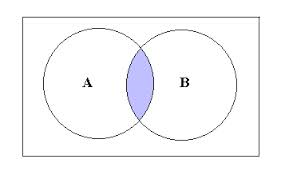#### Some Properties of Operation of Intersection

• Commutative law : $X \cap Y = Y \cap X$
• Associative law : $\left( {X \cap Y} \right) \cap Z = X \cap \left( {Y \cap Z} \right)$
• Law of $\cap$ and $U$ : $\phi \cap X = \phi$ , $U \cap X = X$
• Idempotent law : $X \cap X = X$
• Distributive law : $X \cap \left( {Y \cup Z} \right) = \left( {X \cap Y} \right) \cup \left( {X \cap Z} \right)$

### Difference of set

The difference of two sets $A$ and $B$ is the set $C$ which consists of all those elements which are present in $A$ but not in $B$ . In symbols, we write,
$A - B = \left\{ {x:x \in A{\rm{ \;and\; }}x \notin B} \right\}$

#### Venn Digram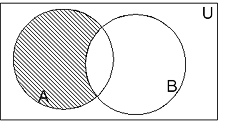#### Some Properties of Operation of Difference

• $A - B \ne B - A$
• The sets $\left( {A - B} \right)$ , $\left( {A \cap B} \right)$ and $\left( {B - A} \right)$ are mutually disjoint sets.

### Compliment of set

Let $U$ be the universal set and $A$ a subset of $U$. Then the complement of $A$ is the set of all elements of U$U$ which are not the elements of $A$. Symbolically, we write $A'$ to denote the complement of $A$ with respect to $U$. Thus,
$A' = \left\{ {x:x \in U{\rm{\; and\; }}x \notin A} \right\}$ ,obviously $A' = U - A$

#### Venn Digram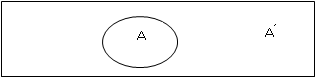#### Some Properties of compliment of sets

1. Complement laws:
• $A \cup A' = U$
• $A \cap A' = \phi$
2. De Morgan's law:
• $\left( {A \cup B} \right)' = A' \cap B'$
• $\left( {A \cap B} \right)' = A' \cup B'$
3. Law of double complementation: $\left( {A'} \right)' = A$
4. Laws of empty set and universal set : $\phi ' = U$ and $U' = \phi$

## Solved Example

Question 1
Let A = { 1, 2, 3, 4, 5, 6}, B = { 2, 4, 6, 8 }. Find
a) A - B
b) B - A
c) A ∩ B
d) A ∪ B
Solution
a) A - B ={1,3,5}
b) B -A ={8}
c) A ∩ B ={2,4,6}
d) A ∪ B={1,2,3,4,5,6,8}

### Quiz Time

Question 1 if set A={1,2,3,4},then how many elements will be present in power set of A
A) 4
B) 16
C) 32
D) 8
Question 2 If P and Q are two sets such that P ∪ Q has 40 elements, P has 12 elements and Q has 42 elements, how many elements does P ∩ Q have ?
A) 54
B) 10
C) 14
D) None of the above
Question 3 which of following is incorrect for set P and Q
A) P ∩ Q= Q ∩ P
B) P ∪ Q= Q ∪ P
C) $\left( {P \cup Q} \right)' = P' \cap Q'$
D) P -Q = Q - P
Question 4 which of the following is incorrect for set A which is subset of Universal set U
A) $A \cap A' = \phi$
B) $\left( {A'} \right)' = A$
C) $A \cup A' = U$
D) $A' = A - U$
Question 5 A = { 1,2,3,4,5} and B ={2,3,4,5} then which of below is false
A) A - B ={1}
B) B -A ={1}
C)A ∪ B ={1,2,3,4,5}
D)A ∩ B=2,3,4,5}
Question 6If A and B are two sets, then A ∩ (A ∪ B) equal
A) A
B) B
C) $\phi$
D) A ∩ B